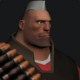# Tutorial : Lighting & Shading

Lighting and Shading is one of the most basic and important things of any rendered scene. During fixed function pipeline days lighting mostly used to be phong material with a directional, point or spot light, but which the support for shader developers are free to implement any kind of light or shading model they want. So lighting and shading isn’t single thing but two different things that are closely related.This tutorial is meant for an introductory step into one of the most interesting (at least for me) and challenging topic of lighting and shading.

## Lighting

Lighting refers to modeling how light behaves in a virtual scene . Most of the time goal is to model the real world lights in a virtual scene as accurately as possible. But there’s a catch our processors (CPU / GPU) are not powerful enough yet to model behaviours of real world lights specially in realtime rendering. So we make some good assumptions and try to simply lights in a virtual scene which led to different lighting models. These lighting model decides the properties of light reaching the surface point.

1. Directional Light – Directional assumes that object or the surface receiving light is either infinitely away or small from the light source so that the difference between the angles of incidence from the light position to various points of the  surface is negligible. So we can assume that light rays reaching the surface are parallel to each other which greatly simplifies the lighting calculation. To describe directional light we need just 2 constants the direction and the color of light. (We also assume the light energy or more appropriately luminous flux is a constant value.)2. Point Light – Point Light assumes that the light source is a single point in the virtual space that emits light energy in all direction or in a uniform spherical region around it. In this case light energy reaching surface point also depends upon the distance between the point and the light source which is proportional to inverse square distance between light source and surface point (called fall off function). Also the light direction is different for different points on the same surface and we have to calculate this vector individual for each vertex or each pixel in case of pixel lighting.  So in case of point lights the intensity of light reaching a surface point depends upon – Distance between surface point and the light source calculated for each point. The light direction vector from surface point in question to light source calculated for each point. The light color constant for a light.3. Ambient Light – The above two are the examples of direct lights trying to model real world counterparts. The ambient light on the other hand is not a light in itself but the cheapest way to model indirect lighting or the real world behaviour of light bouncing of the surfaces and illuminating the surroundings. In this model a constant light color value is scaled by the surface albedo is added to the shading equation to model indirect lighting.(notice the cube is invisible because of flattening side effect of ambient light)
4. There are many more types of light like area lights, spot light etc. What I have mentioned here is the most basic types of light that can be used to lit virtual scenes at cost of very small performance hit on today’s hardware.(Warn light – a way to model spot light)

Appearance of an object in real world depends on how light is reflected by the object (ignoring transmittance for simplicity). Lighting models describes how light reaches a surface and the job of shading models or shading equation is to compute the outgoing light value along the view direction depending on the material properties of the surface. Shading models try to emulate the real world object surfaces like metals , plastics ,etc.

So in simple terms a shading equation can be given as –

Outgoing Light = Reflectance function * Incoming Light value at surface point to be shaded

A function that describes how light is reflected by the surface given the incoming light direction and the outgoing direction (most of the time the view direction) is known as Bidirection Reflectance Distribution Functions (BRDF) .

Some materials scatter light evenly in all directions and are called Diffuse Materials.  Simple BRDF to model such materials is  Lambertian BRDF,

whereis the intensity of the diffusely reflected light (surface brightness),is the color andis the intensity of the incoming light.

Another class of materials called specular materials reflect light only in mirror direction. Generally a real world material is neither purely diffuse or purely specular so both models are combines to emulate real world materials. One simple BRDF used to model such materials invented by developed by Bui Tuong Phong in 1973 is Phong BRDF.

In advance shading models the directional dependence of reflective properties of a surface materials are also considered and are classified as Isotropic and Anisotropic Materials. One of the BRDFs to model such materials is WARD BRDF.After experimenting with some basic light models the right direction will be to read tests related to physically based rendering. Those texts get more technical with complex equations on every page so this was ment to be a simple basic tutorial to get started on things and also to give some simple models to work with. Also shaders for these BRDFs and lighting models can easily be founded on google. Here are a couple of very nice references on this subject (these books assume you are comfortable with shader based pipeline) –